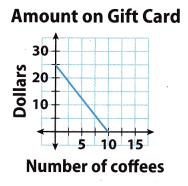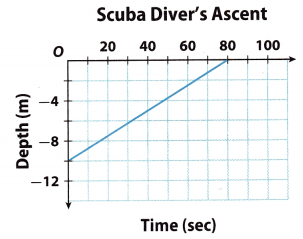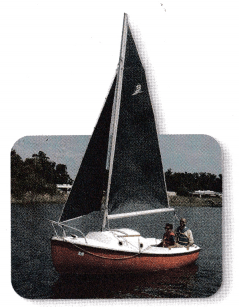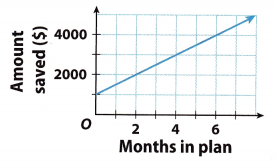# Texas Go Math Grade 8 Lesson 5.1 Answer Key Writing Linear Equations from Situations and Graphs

Refer to our Texas Go Math Grade 8 Answer Key Pdf to score good marks in the exams. Test yourself by practicing the problems from Texas Go Math Grade 8 Lesson 5.1 Answer Key Writing Linear Equations from Situations and Graphs.

## Texas Go Math Grade 8 Lesson 5.1 Answer Key Writing Linear Equations from Situations and Graphs

Essential Question
How do you write an equation to model a linear relationship given a graph or a description?

Explore Activity
Writing an Equation in Slope-intercept Form

Greta makes clay mugs and bowls as gifts at the Crafty Studio. She pays a membership fee of $15 a month and an equipment fee of$3.00 an hour to use the potter’s wheel, table, and kiln.Write an equation in the form y = mx + b that Greta can use to calculate her monthly costs.

A. What is the input variable, x, for this situation?
What is the output variable, y for this situation?

B. During April, Greta does not use the equipment at all.
What will be her number of hours (x) for April? ______
What will be her cost (y) for April? ______
What will be the y-intercept, b, in the equation? _____

C. Greta spends 8 hours in May for a cost of $15 + 8($3) = ____
In June, she spends 11 hours for a cost of _________________
From May to June, the change in x-values is _______________
From May to June, the change in y-values is _______________
What will be the slope, m, in the equation? ______

D. Use the values for m and b to write an equation for Greta’s costs in the form y = mx + b: __________________

Reflect

Question 1.
What does the value of the slope represent in this context?
The slope represents the rental for each DVD borrowed.

Describe the meaning of the y-intercept.
The y-intercept represents the one-time membership fee.

Question 3.
The cash register subtracts $2.50 from a$25 Coffee Café gift card for every medium coffee the customer buys. Use the graph to write an equation in slope-intercept form to represent this situation.slope = $$\frac{0-25}{10-0}$$ = $$\frac{-25}{10}$$ = -2.5 Finding the slope using given points by Slope(m) = (y2 – y1) ÷ (x2 – x1) where (x2, y2) = (0, 25) and (x1, y1) = (10, 0)
y-intercept (b) = 25 From the graph
y = -2.5x + 25 Substituting the value of slope (m) and y-intercept in slope-intercept form:
y = mx + b
y = -2.5x + 25

Reflect

Question 4.
Without graphing, tell whether the graph of this equation rises or falls from left to right. What does the sign of the slope mean in this context?
Whether the graph is ascending or descending depends on the sign of the slope. If the slope is positive then the graph is increasing, because we get the second point by counting upwards from the starting point (y-intercept, b) for the number written in the slope numerator. If the slope is negative then the graph is descending, because we get the second point by counting down from the starting point (y-intercept, b) for the number written in the slope numerator.

Lesson 5.1 Writing Linear Equations from Situations and Graphs Question 5.
Hari’s weekly allowance varies depending on the number of chores he does. He received $16 in allowance the week he did 12 chores, and$14 in allowance the week he did 8 chores. Write an equation for his allowance in slope-intercept form. _______
Input variables: Number of chores
Output variables Weekly allowance Identify the input and output variables.
(12, 16) Write the information given in the problem as ordered pairs.
(8, 14)
Slope = $$\frac{16-14}{12-8}$$ = $$\frac{2}{4}$$ = $$\frac{1}{2}$$ Finding the slope using given points by slope (m) = (y2 – y1) ÷ (x2 – x1)

14 = $$\frac{1}{2}$$(8) + b Substituting the value of slope (m) and (x, y) in the slope-intercept form to find y-intercept (b):
14 = 4 + b y = mx + b
b = 14 – 4 = 10
y = $$\frac{1}{2}$$x + 10 Substituting the value of slope (m) and y-intercept in slope-intercept form.

Question 1.
Li is making beaded necklaces. For each necklace, she uses 27 spacers, plus 5 beads per inch of necklace length. Write an equation to find how many beads Li needs for each necklace. (Explore Activity)
a. input variable: _____________________________________________
Input variable: The length of the necklace in inches.

b. output variable: _____________________________________
Output variable: The total number of beads in the necklace.

c. equation: ______________
Equation:
y = 5x + 27

Question 2.
Kate is planning a trip to the beach. She estimates her average speed to graph her expected progress on the trip. Write an equation in slope-intercept form that represents the situation. (Example 1)Choose two points on the graph to find the slope.
m = $$\frac{y_{2}-y_{1}}{x_{2}-x_{1}}$$ = _______
Read the y-intercept from the graph: b = ______________
Use your slope and y-intercept values to write an equation in slope-intercept form. _________________________
m = $$\frac{y_{2}-y_{1}}{x_{2}-x_{1}}$$ = $$\frac{0-300}{5-0}$$ = $$\frac{-300}{5}$$ = -60
Finding the slope using given points (x2, y2) = (0, 300) and (x1, y1) = (5, 0)
y-intercept (b) = 300 Read from the graph (when x = 0)
y = -60x + 300 Substituting the value of slope (m) and y-intercept in slope-intercept form:
y = mx + b

Texas Go Math Grade 8 Writing Linear Equations from Graphs 5.1 Answer Key Question 3.
At 59°F, crickets chirp at a rate of 76 times per minute, and at 65°F, they chirp 100 times per minute. Write an equation in slope-intercept form that represents the situation. (Example 2)
Input variable: _____________________
Output variable: __________________________
m = $$\frac{y_{2}-y_{1}}{x_{2}-x_{1}}$$ = __________
Substitute in y = mx + b: _______________ + b; __________ = b
Write an equation in slope-intercept form. ___________________
To determine the equation in slope intercept form y = mx + b that represents the situation At 59° F crickets chirp at a rate of 76 times per minute, and at 65° F, they chirp 100 times per minute, let us follow the following steps.

First, let us identify the input and output variables. The input variable x is the temperature while the output variable y is the number of chirps per minute.

Second, let us write the ordered pairs.
at 59 °F, crickets chirp at a rate of 76 times per minute:(59, 76)
at 65 °F, crickets chirp at a rate of 100 times per minute:(65, 100)
x1 = 59°
y1 = 76
x2 = 65°
y2 = 100

Third, let us determine the slope in using the formula
m = $$\frac{y_{2}-y_{1}}{x_{2}-x_{1}}$$
Substituting the values of x1, y1, x2 and y2.
m = $$\frac{100-76}{65-59}$$
m = $$\frac{24}{6}$$
m = 4

Fourth, let us find the value of the y-intercept b. Using the variables and the slope 4, we can have two equations
for us to find b, these are
76 = 4 • 59 + b
and
100 = 4 • 65 + b
We can use any of these two to determine the value of b, that is
100 = 4 • 65
100 = 260 + b
b = 100 – 260
b = -160

Now, let us form the equation in slope-intercept form, having the slope m = 4 and y-intercept b = -160, that is
y = mx + b
y = 4x – 160
see the explanation

Essential Question Check-In

Question 4.
Explain what m and b in the equation y = mx + b tell you about the graph of the line with that equation.
m indicates the steepness of the line and whether the graph is rising or falling (left from right). b indicates the point where the line crosses the y-axis.

Question 5.
A dragonfly can beat its wings 30 times per second. Write an equation in slope-intercept form that shows the relationship between flying time in seconds and the number of times the dragonfly beats its wings.
Input variable: Flying time in seconds Identify the input and output variables.
Output variable: Number of times the dragonfly beats its wing
y = 30x Substituting the value of slope (m) and y-intercept in slope-intercept form.
where m = 30 times per second and b = 0

Go Math 8th Grade Pdf Lesson 5.1 Equations and Their Graphs Question 6.
A balloon is released from the top of a platform that is 50 meters tall. The balloon rises at the rate of 4 meters per second. Write an equation in slope-intercept form that tells the height of the balloon above the ground after a given number of seconds.
The problem is asking for the equation that tells the height of the balloon above the ground after a given number of seconds in slope-intercept form.

The statement that the balloon is released from the top of a platform that is 50 meters tall and rises at the rate of 4 meters per second
Let
y be the height of the balloon above the ground, x be the number of seconds, and 4s be the rate of the balloon as it rises per second.

Using the representation and the given, we should equate the height of the balloon above the ground y to the rate of the balloon as it rises per second 4x added to 50 which is the height of the platform, which gives us the equation,
y = 4x + 50

The graph shows a scuba diver’s ascent over time.Question 7.
Use the graph to find the slope of the line. Tell what the slope means in this context.
______________________
First, we choose two points on the graph to find the slope. We substitute (0, -10) for (x1, y1) and (80, 0) for (x2, y2)Therefore, the slope equals to 0.125 means that the scuba driver ascends 0.125 meters each second.

Question 8.
Identify the y-intercept. Tell what the y-intercept means in this context.
The starting point on the graph is (0, -10), i.e. (0, b). So the value of the y-intercept is -10. Reading from the graph, the y-intercept is the value for the depth (in meters) from which the scuba diver started to swim to the surface.

Question 9.
Write an equation in slope-intercept form that represents the diver’s depth over time.
From the given graph, find two points that will help you calculate the slope.
I’ll take (0, -10) for (x1, y1) and (80, 0) for (x2, y2).
Thus:The starting point on the graph is (0, -10), ie. (0, b). So the value of the y-intercept is -10. → b = -10

The standard form of the linear equation is y = mx + b, so include the obtained values of the slope and y-intercept.
y = $$\frac{1}{8}$$x – 10

Texas Go Math Grade 8 Pdf Lesson 5.1 Answer Key Question 10.
The formula for converting Celsius temperatures to Fahrenheit temperatures is a linear equation. Water freezes at 0°C, or 32°F, and it boils at 100°C, or 212°F. Find the slope and y-intercept for a graph that gives degrees Celsius on the horizontal axis and degrees Fahrenheit on the vertical axis. Then write an equation in slope-intercept form that converts degrees Celsius into degrees Fahrenheit.
To determine the equation in slope intercept form y = mx + b that converts degrees Celsius into degrees. Fahrenheit, let us follow the following steps by finding the slope and y-intercept form given that the water freezes at 0°C or 32°F and it boils at 100°C or 212°F

First, let us identify the input and output variables. The input variable x is the temperature in degrees Celsius while the output variable y is the temperature in degrees Fahrenheit

Second, let us write the ordered pairs.
water freezes at 0°C or 32°F:(0, 32)
water boils at 100°C or 212°F:(100, 212)
x1 = 0
y1 = 32
x2 = 100
y2 = 212

Third, let us determine the slope ni using the formula
m = $$\frac{y_{2}-y_{1}}{x_{2}-x_{1}}$$
Substituting the values of x1, y1, x2, and y2
m = $$\frac{212-32}{100-0}$$
m = $$\frac{180}{100}$$
m = 1.8

Fourth, let us find the value of the y-intercept b. Using the variables and the slope 4, we can have two equations for us to find b, these are
32 = 1.8 . 0 + b
and
212 = 1.8 . 100 + b
We can use any of these two to determine the value of b, that is
212 = 1.8 . 100 + b
212 = 180 + b
b = 212 – 180
b = 32
Now, let us form the equation in slope-intercept form, having the slope m = 1.8 and y-intercept b = 32, that is
y = mx + b
y = 1.8x + 32

See the explanation.

Question 11.
The cost of renting a sailboat at a lake is $20 per hour plus$12 for lifejackets. Write an equation in slope-intercept form that can be used to calculate the total amount you would pay for using this sailboat.To determine the equation in slope-intercept form to calculate the total amount you would pay for using a sailboat given that the cost of renting a sailboat at a lake is $20 per hour plus$12 for life jackets, let us represent the different variables in the problem.

Let y be the total cost you would pay for using a sailboat, x be the number of hours you would use the sailboat, $20 which is the cost of renting a sailboat per hour be the slope, and$12 is the cost of lifejackets be the y-intercept.

Now using the slope-intercept form, we can have the equation by substituting the value of where m = 20 and b = 12, that is
y = mx + b
y = 20x + 12

The graph shows the activity in a savings account.Question 12.
What was the amount of the initial deposit that started this savings account?
Reading from the graph, the y-intercept is the starting point: (0, 1000), so the value for the y-intercept is 1000. Thus,
b = 1000\$.
So that value is the amount of the initial deposit that he started with his savings account.

Find the slope and y-intercept of the graphed line.
From the given graph, find two points that will help you calculate the slope.
I’ll take (0, 1000) for (x1, y1) and (6, 4000) for (x2, y2).
Thus:
m = $$\frac{4000-1000}{6-0}$$ = $$\frac{3000}{6}$$ = 500
The starting point on the graph is (0, 1000), i.e. (0, b). So value of the y-intercept is 1000. → b = 1000

Question 14.
Write an equation in slope-intercept form for the activity in this savings account.
The standard form of the linear equation is y = mx + b. In previous exercise we got values for the slope and y intercept → m = 500, b = 1000. Include the obtained values of the slope and y-intercept into the equation.
y = 500x + 1000

Let us describe the equation: y represents the total amount of saved money, x represents the time of collecting money (in months), the slope represents the money saved during x months and y-intercept represents the amount with which he started to save.

Question 15.
Explain the meaning of the slope in this graph.
The slope represents the amount of money saved in dollars per month in plan.

Texas Go Math Grade 8 Lesson 5.1 H.O.T. Higher Order Thinking Answer Key

Question 16.
Communicate Mathematical Ideas Explain how you decide which part of a problem will be represented by the variable x, and which part will be represented by the variable y in a graph of the situation.
Explanation A:
You need to analyze the problem to find out which part depends on another. That dependent part will be represented by variable y, and the independent part by x.
You need to analyze the problem to find out which part depends on another.

Explanation B:
Variable x is the input, which represents the variable that affects the other variable when changed and is independent.
Variable y is the output, which represents the resulting variable or the variable that is dependent on the other.

Question 17.
Represent Real-World Problems Describe what would be true about the rate of change in a situation that could not be represented by a graphed line and an equation in the form y = mx + b.
The rate of change would not be constant. Using different pairs of points in the slope formula would give different results.

Lesson 5.1 Writing Linear Equations from Situations and Graphs Answer Key Question 18.
Draw Conclusions Must m, in the equation y = mx + b, always be a positive number? Explain.